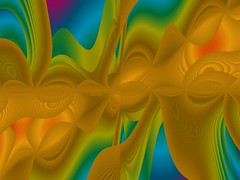# Genetic Art – Part 4

After some research into binary expression trees, infix, prefix and postfix I was able to create a new Genetic Art 3 mode for Visions Of Chaos.

Basing the image formulas on expression trees allows far more complex images to be bred using mutations, combinations and crossover.

Too see a full range of images this new Genetic Art method is capable of see this flickr set.

Here are a few thumbnails and the formulas that create them.((((y+x)*arctan((x*round(pi))))%((((y+x)*arctan((y/int(pi))))-cos((x+5.4)))%(((y%y)-y)%(2.7*4.5))))+((((y+x)*arctan((y/int(pi))))-cos((x+5.4)))%(((y%y)-y)%(2.7*4.5))))(((pi%4.2)*arctan(((x*y)+sin((y*tan(7.2))))))*((((pi%4.2)*arctan(((x*y)+sin((y*tan(7.2))))))%(((y-sin(y))%(x*sqrt(pi)))-(((1.2-pi)%sgn((x/4.2)))*abs((x+y)))))-((pi%4.2)*arctan(((x*y)+sin((y*tan(7.2))))))))(((((1.3/0.9)+sin((y*x)))%(((1.3/0.9)+sin((y*x)))%(((y*0.5)%6.5)-cos((x%(y-sqr(pi)))))))+((((1.3/0.9)+sin((y*x)))-(((1.3/0.9)+sin((y*x)))%(((y*0.5)%6.5)-cos((x%(y-sqr(pi)))))))*(((1.3/0.9)+sin((y*x)))%(((y*0.5)%6.5)-cos((x%(y-sqr(pi))))))))+((((1.3/0.9)+sin((y*x)))%(((1.3/0.9)+sin((y*x)))%(((y*0.5)%6.5)-cos((x%(y-sqr(pi)))))))+((((1.3/0.9)+sin((y*x)))-(((1.3/0.9)+sin((y*x)))%(((y*0.5)%6.5)-cos((x%(y-sqr(pi)))))))%(((y+round(pi))%(x+cos(y)))+int(((x/7.4)*(y-(7.4*sin(7.2)))))))))

There is also a high-def video of Genetic Art animations here. The animations change the digit nodes in the expression tree slightly over each frame.

Jason.

# Knowledge must be free

The human species advances from building on prior knowledge and sharing knowledge.

The Internet is amazing for this (in the words of Lisa Simpson “Bart, The Internet is more than a global pornography network!”). Being able to access and search for information on any topic has been invaluable for myself when writing Visions Of Chaos.

But recently (actually not so recently as it has happened for a while now) when trying to access a research paper on a specific subject you get sent to some registration page that gives you a quick abstract overview and then wants \$25 to be able to download the paper. This sucks and should be outlawed.

Luckily if you then search on the title of the paper you are after you can usually find a cached copy somewhere, but not always.

There are still good indexes and search engines out there specifically for scientific related papers like CiteSeer which caches copies of the papers in PDF, PS etc. For anyone into research into any science related field CiteSeer is brilliant.

Knowledge needs to be free and accessible by anyone.

Jason.

# Genetic Art – Part 3

picbreeder is an amazing example of genetic art online that allows anyone to evolve existing images or start from scratch and evolve their own. See here for some great examples.

picbreeder is the first genetic art system I have seen that has results beyond the usual abstract output of genetic art software and can actually produce images that “look like something” (even though in some cases it is clearly Pareidolia). Here are a few example images (there are thousands more in their gallery);The back end uses neural networks that mutate and breed that control the images. The results are very inspiring.

After a long waste of time most interesting experiment with the website these two are examples of what I evolved.If you want to be distracted (in a good way) for a while give it a try. Everyone that contributes increases the gene pool of images. So be your own God artist.

There is also a nice collection of genetic art links here.

Jason.